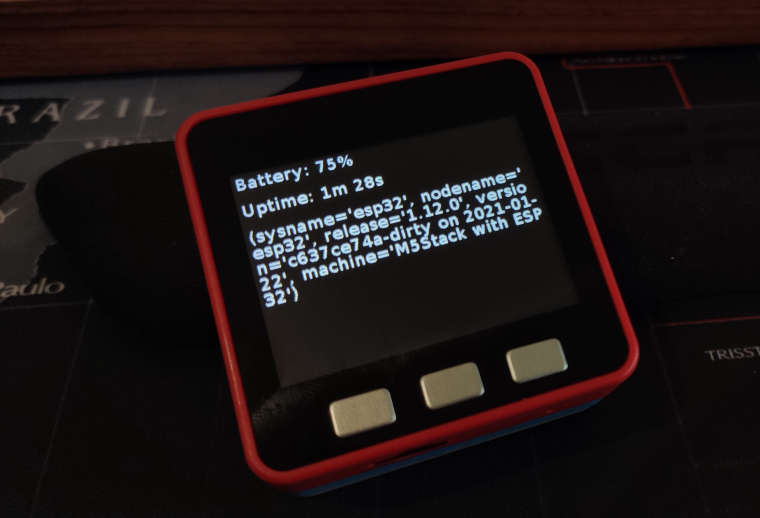•``````from m5stack import *
from m5ui import *
from uiflow import *
import time
import uos

setScreenColor(0x000000)

vstart = None
vsysinfo = None
vsecs = None
vmins = None
vtimetext = None

import math

lcd.setBrightness(10)
vstart = time.ticks_ms()
vsysinfo = uos.uname()
lb_sysinfo.setText(str(vsysinfo))
while True:
battery.setText(str((str(((str('Battery: ') + str((power.getBatteryLevel()))))) + str('%'))))
vsecs = round(((time.ticks_ms()) - vstart) / 1000)
vmins = math.floor(vsecs / 60)
vtimetext = (str((vsecs % 60)) + str('s'))
if vmins != 0:
vtimetext = (str(((str(vmins) + str('m ')))) + str(vtimetext))
vtimetext = (str('Uptime: ') + str(vtimetext))
uptime.setText(str(vtimetext))
wait_ms(2)
``````

• Interesting, when executing on a M5Stack Core2 via REPL I get an error message:

``````Traceback (most recent call last):
File "<stdin>", line 21, in <module>
AttributeError: 'TFT' object has no attribute 'setBrightness'
``````

• @mb

Different code...

Core:
`lcd.setBrightness(10)`

Core2:
`screen.set_screen_brightness(10)`

• I would prefer to have a stable API for all devices. Current situation makes re-use of code complicated.

Someone could write a wrapper API to cover the differences ;-)xkcd comic

• I'm trying to push for a unified API but the problem is that some of the devices are slightly different and require slightly different commands.